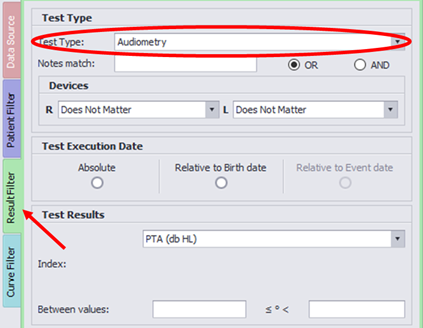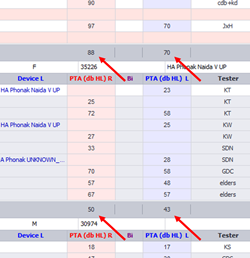After executing a query, Audiqueen Analytics calculates the average of the results.  It is important to understand Audiqueen’s working principles.Audiqueen shows how  many subjects and results were found after executing the query and how many have been selected
for the calculation of the averages (red arrow).  It also shows the average results for the measurements from the right, the left and both ears (red circle).

#### When does Audiqueen calculate an average?

Audiqueen only calculates an average if a Test Type is selected in the Result Filter. It would make no sense to calculate an averages of results of different test types.#### What does I and C stand for?

In the results grid, the column headers can be either R/Both/L or I/Both/C.  I stands for ipsilateral and C for contralateral and these are used if the event used in the Patient Filter is ear-specific.  This is typically the case for surgical interventions.

#### Grouping

Audiqueen tries to group results if possible.

• The first grouping variable is the event (which would typically be a surgical intervention).  So if an event is selected in the Patient Filter, Audiqueen calculates the average test result per event.
• The second grouping variable is the patient’s ear.  So if an event occurs for the right ear and another one for the left ear, Audiqueen calculates the average results for each ear separately. Also, if more events occur at the same side, Audiqueen calculates the average result per event, then the average for each ear.  Each patient thus can have a maximum of 3 averages, 1 for the right side (or the ipsilateral side), 1 for the left side (or contralateral side) and 1 for results linked to both sides.

Hence, the average result for a patient's otosclerosis operation (as an example) is an average of averages, namely the average of the average results of each intervention.  If the patient underwent 2 otosclerosis interventions at the right ear, and one at the left ear, Audiqueen calculates the average result for the first intervention (right) and the average result for the second intervention (right) and the average of the single intervention (left).  So there are 3 'ipsilateral' otosclerosis results.  Then Audiqueen calculates the average of these 3 average results.  This is the Ipsilateral otosclerosis result for this patient.

By default, Audiqueen does not show these averages per patient.  You can check the corresponding checkbox in the Analytics window.When checking the checkbox, Audiqueen displays the average values per patient.

For the final average (shown in the header of the results window), Audiqueen calculates the average of these averages, so it calculates 1 average for the right (or ipsilateral) ear, one for the left (or contralaterals) ear and one for both ears.

#### How does Audiqueen treat ‘no response’ points?

It can occur in audiometry that a ‘no response threshold is set’.  On the audiogram this is shown as a point, for instance at 80 dB, with a ‘no response’ symbol.  If this point is a component of the calculated index, Audiqueen takes its value, calculates the index, and displays the calculated index preceded by the > sign.

For example: if the PTA is calculated based on 3 thresholds, and 2 thresholds are 75 dB and the third threshold is ‘no response at 90 dB’, Audiqueen displays the index as ‘> 80’.

If such an index is used for the calculation of an average of indices, Audiqueen takes the value, in the example case it would take 80 for the calculation of the average PTA of several audiograms.

#### How does Audiqueen treat N/A points?

It may occur that certain points have not been measured, for instance an audiometric threshold at 2000 Hz, or a speech audiometric score at 50 dB.  If such point is required to calculate the index, Audiqueen displays N/A in the index field.  Such an N/A is not used for the calculation of an average index.#### Can I overrule Audiqueen’s choices?

If you don’t agree with Audiqueen’s choices, there are 2 options left:

• You can manually unselect test results from the grid.  In this case, the result will not be used for the calculation of averages.• You can export all raw data to an excel sheet and decide for yourself how to treat them.